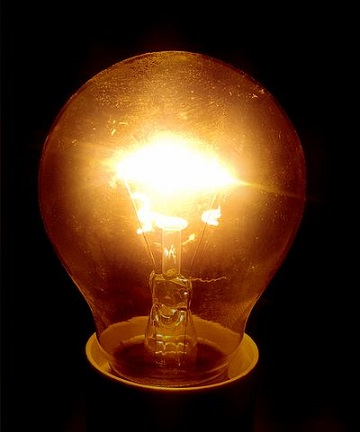# Conductor resistanceFigure 1. The filament of an incandescent lights up because of the resistance provided by the conducting wire.

Conductor resistance is a property of a conductor at a specific temperature, and it is defined as the amount of opposition there is to the flow of electric current through a conducting medium. The resistance of a conductor depends on the cross sectional area of the conductor, the length of the conductor, and its resistivity. It is important to note that electrical conductivity and resistivity are inversely proportional, meaning that the more conductive something is the less resistive it is.

The resistance of a conductor can be calculated at a temperature of 20°C using:

where:

• is the resistance, in ohms (Ω)
• is the resistivity of the material in ohm meters (Ωm)
• is the length of the conductor in meters (m)
• is the cross-sectional area of the conductor in meters squared (m2)

This formula tells us that the resistance of the conductor is directly proportional to and , and inversely proportional to . Since the resistance of some conductor, such as a piece of wire, depends on collisions within the wire itself, the resistance depends on temperature. With increasing temperature, the resistance of the wire increases as collisions within the wire increase and "slow" the flow of current. The amount of change is determined by the temperature coefficient. A positive temperature coefficient results in resistance increasing with temperature whereas a negative temperature coefficient results in a decreasing resistance with an increasing temperature. Since conductors typically display an increased resistivity with temperature increase, they have a positive temperature coefficient. The most common types of resistors are variable resistors and fixed resistors.

By utilizing the resistance of a conductor, light can be created in an incandescent light bulb. In an incandescent light bulb there is a wire filament that is a certain length and width, thus providing a certain resistance. If this resistance is just right, the current flowing through the wire is slowed just enough, without stopping as a result of too much resistance, that the filament heats up to the point that it glows.

For more on conductor resistance, see HyperPhysics.

## PhET: Resistance in a wire

The University of Colorado has graciously allowed us to use the following Phet simulation. Explore the simulation to see how resistance of a conductor changes depending on geometry and resistivity:

## For Further Reading

For further information please see the related pages below:

## Authors and Editors

Bethel Afework, Jordan Hanania, Ellen Lloyd, Kailyn Stenhouse, Jasdeep Toor, Jason Donev
Last updated: June 4, 2018
Get Citation